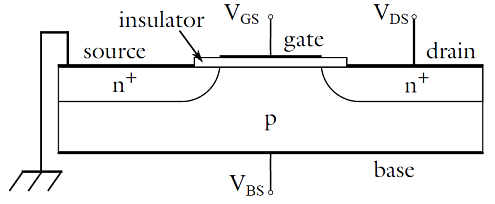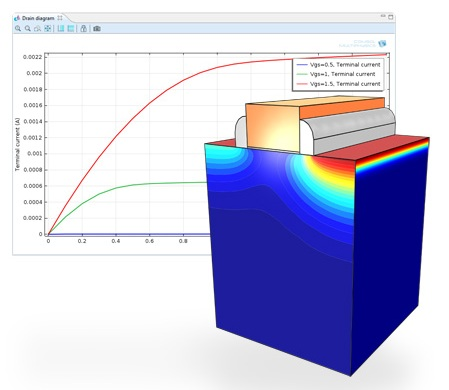# 石墨烯革命：第五部分

2013年 5月 8日

### 什么是 MOSFET？

MOSFET 的基本思路是施加栅极电压来控制漏源电阻，进而控制漏电流（见下图）。在某个栅源电压 (VGS)以及较低的漏源电压 (VDS) 下，漏电流几乎线性依赖于 VDS。VDS 增加时，漏电流将达到饱和。饱和程度依赖于栅源电压，切换时间取决于半导体的迁移率。半导体材料的迁移率越高，接通及断开电流越快。### 半导体物理学

\begin{aligned}
\frac{\partial n}{\partial t}&=\frac{1}{q}\nabla \cdot \mathbf{J_{n}}-R_n \\
\frac{\partial p}{\partial t}&=-\frac{1}{q}\nabla \cdot \mathbf{J_{p}}-R_p \\
\nabla \cdot{} \left(\epsilon \nabla V\right) &= -q(p-n+N_D^+-N_A^-)
\end{aligned}

### 我们全新的半导体模块### 扩展阅读

#### 评论 (1)

##### 留言2016-03-05